# Luke Clemens March 6, 2000

Have you ever spent a rainy day spelunking through a fractal? Exploring a fractal is very similar to wandering through a cave and marveling at stalagtites and crystal structures. Caves, trees, plants, clouds, water, and even matter itself exhibits properties that are fractal in origin. A fractal is a mathematical formula that generates intricate patterns with relitively little programming code. Most fractals contain miniature versions of themselves, sometimes hidden away, but in some cases they are very obvious. Another common characteristic is the use of recursion. Looking at a fractal, we can see that the location of a particular point is not random, rather, it is affected by the placement of previous points. This is easily seen in a tree by noting that the location of a specific twig in space is affected by the location of the larger branch that it is attached to, which is in turn affected by the even larger tree trunk. Recursion defines the present through the past. One of the first fractals to be discovered was the Mandelbrot set by French Mathematician Benoit B. Mandelbrot in 1980. Performing the necessary calculations for a Mandelbrot picture is nearly impossible by hand, but with the efficiency and accuracy of a computer, the task is greatly simplified. The material presented here will give the reader a glimpse into the Mandelbrot equation along with an overview of the code implementation.

Before we examine the fundamental Mandelbrot equation in depth, we must be sure that we have an understanding of imaginary numbers and their basic operations. After a quick review, we'll be ready to move on to the real core of the material.

If we were try and solve the equationwe would find that it does not have a solution using the conventional algebraic system and real numbers. To remedy this, mathemeticians have devised a system of complex numbers which simply substitutesfor i. With this sytem, we can create complex numbers in the form a + ib. Here "a" is the real part, and "ib" is the imaginary part. For example, in the above equation,, we can produce the following by solving for x:Note that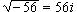,,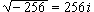, ect... To add/subtract two complex numbers, we add/subtract the real part of the first, to the real part of the second, and the imaginary part of the first to the imaginary part of the second. Multiplication of complex numbers is slightly tougher, but plays an important role in creating the Mandelbrot set, so close attention is necessary if it's not already burned in your head. There are two methods to do this, so take your pick. The first method is faster and easier to translate to computer language, while the second is not computer ready, but is a solid and proven way to check your answer for the first method.

Method 1: According to Berman page 480, (a+bi)(c+di) = (ac-bd) + i(ad+bc) . Using this formula we can solve the example (5+2i)(3-4i) with the following:

(5+2i)(3-4i) = 5(3) - 2(-4) + i[5(-4) + 2(3)]
= 15 + 8 + i(-20+6)
= 23 - 14i

Method 2: First multiply the two complex number together exactly as you would do (a + bx)(c+dx) using the FOIL method from gradeschool -- you know... first outside inside last. Following our example above:

(5+2i)(3-4i) = 5(3) - 4(5)i + 2i(3) - 8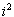-----note that=*= -1
= 15 + 20i + 6i - 8(-1)
= 15 + 8 + i(-20 + 6)
= 23 - 14i

Now that imaginary numbers are out of the way, we can move into the heart of the Mandelbrot set. The Mandelbrot function is a first degree non-homogeneous recurrence relation. Because it is a recurrence relation, the Madelbrot set, like other fractals, posseses the property of self-similarity. This means that it contains miniature versions of itself within the bigger picture. Brace yourself, because the Mandelbrot equation in its raw form is about to be revealed!

The Mandelbrot set is defined as:

All points c, where c is a complex variable, such that the iteration(the complex number "c" is a constant for each orbit)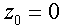(base case)

does not escape to infinity.

All recursive functions have what is known as an orbit --a list of successive iterates from a starting point. To find the orbit of a function, the function is iterated a number of times until it can be determined if it goes to infinity or not. For example, the orbit of "f(x) = x" escapes to infinity --since as x increases, the image, "x", heads toward infinity. Some orbits go to infinity, some to zero, and others to an arbitrary constant. A periodic orbit occurs when an orbit tends to more than one value. Some functions have an orbit that is unstable and goes to seemingly random values. These orbits are the ones that are the most interesting, and the ones that create fractals such as the Mandelbrot set. Yup. The 'purdy' pictures.

Through examining an example of an orbit using imaginary numbers, a thorough understanding of the process can be attained. Let's pretend that c = 1 - i. This being the case, our equation now becomes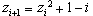. Now we're going to iterate it until we decide that it is either going to infinity or going somewhere else. You will need to refer back to the equation for multiplying imaginary numbers, (stated below for convenience):

(a+bi)(c+di) = (ac-bd) + i(ad+bc) (imaginary multiplication)(our original equation)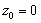(base case)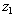= (0+0i)+ 1 - i=
1 - i
==========================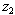=(1-i)+ 1 - i=(1-1i)(1-1i) + 1 - i           a=1, b=-1, c=1, d=-1=
1(1) - -1(-1) + i[1(-1) + (-1)(1)] + 1 - i=
1 - 1 + i[-2] + 1 - i= 1 - 2i - i= 1 - 3i
==========================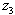= (1-3i)+ 1 - i= (1-3i)(1-3i) + 1 - i          a=1, b=-3, c=1, d=-3= 1(1) - -3(-3) + i[1(-3) + -3(1)] + 1 - i= 1 - 9 + -6i + 1 - i= -7 - 7i
==========================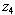= (-7 - 7i)+ 1 - i= (-7 - 7i)(-7 - 7i) + 1 - i          a=-7, b=-7, c=-7, d=-7= (-7)(-7) - (-7)(-7) + i[-7(-7) + -7(-7)] + 1 - i= 49 - 49 + i(49 + 49) + 1 - i= 1 + 98i - i= 1 + 97i

Here is a summary of the resulting equation:
Figure A

 Iteration Number (i) Value of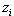0 0 + 0i 1 1 - i 2 1 - 3i 3 -7 - 7i 4 1 + 97i

We can make a guess that in this example, our orbit will escape toward infinity. For a different value of "c", however, it may escape to zero, or possibly some other value. Since this orbit did escape to infinity, we would say that it does NOT belong to the Mandelbrot set. So how is the computer to decide when it escapes to infinity? If we know how far the point is from the origin, we can check to see if its distance from the origin is greater than some value between 3 and 5. As to why 3 is the minimum, you'll have to look elswhere. There's a proof for it, but it's long and complicated so we're going to have to take it as an "if you say so Calvin". 5 is the upper bound because some PCs will choke if it's much higher and visual quality gains become inperceptable on a computer monitor at that level. Later we will refer to this variable as big "R", and for our code we'll use 4 as its value just in case someone out there's still hanging on to the ol' 386. It won't affect image quality, (the author tried it), although setting it lower than 3 will produce some interesting results.

Imagine for a second, a graphing coordinate system in which the x axis is the real axis, and the y axis is the imaginary axis. On this system, a + bi can be mapped to (a,bi) -- basically (real, imaginary). The real and imaginary limits will both be -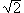to. Did you imagine something like the graph below? It is formally called an Argand Plane.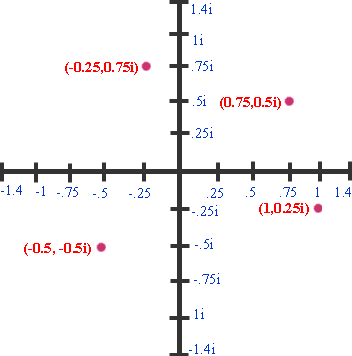Perhaps the trickiest part of the procedure is deciding which values of the complex variable "c"we should use. This is where your souped up high-octane megahertz stomping PC with an attitude comes in. We simply set up a nested "for" loop and iterate through each pixel to determine whether the pixel is part of the set, (in other words see if its orbit does not escape to infinity). Essentially, the computer will loop through each pixel and ask, "Are you part of the Mandelbrot set?" The real part of "c" is set to -1.4 + (x(2.8/screen_width)) and the imaginary part is set to -1.4 + (y(2.8/screen_height)). This converts the the particular pixel on the screen (x,y) to the scale -1.4 to1.4 for the Argand Plane's (real,imaginary) set of points. Note thatis roughly 1.4. Time for some hacking! Setting up this code for a 400x400 pixel screen procedes as follows:

// First we will need a struct that holds a real part and an imaginary part.
struct complexNumber {
double real;     // use doubles because more precise values will allow more accurate pictures
double imaginary;
};

complexNumber c;      // Declare a complex number named c.
int x, y;       // Iterators for the x and y coordinates on the screen

for (x = 0; x < 400; x++) {
for (y = 0; y < 400; y++) {
c.real = -1.4 + (y * (2.8/400));       // convert each pixel to the argand plane (pixel-to-argand conversion)
c.imaginary = -1.4 + (x * (2.8/400));
}
}

It is probably easiest if you create a function for adding complex numbers and another function for multiplying. They follow the same rules as stated earlier.

complexNumber complexAdd(complexNumber left, complexNumber right) {
// (a+bi) + (c+di) = (a + c) + i(b + d)
ComplexNumber answer;       // declare a local complex number
}

complexNumber complexMultiply(complexNumber left, complexNumber right) {
ComplexNumber answer;       // declare a local complex number
// (a+bi)(c+di) = (ac-bd) + i(ad+bc)

answer.real = (left.real * right.real) - (left.imaginary * right.imaginary);
answer.imaginary = (left.real * right.imaginary) + (left.imaginary * right.real);
}

Now that we have complex number addition and multiplication in our tool-chest, we can concentrate on finding orbits of the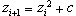equation. Thanks to the pixel-to-argand conversions, c will now be defined. A new equation exists with a new value of c for each pixel. We could store each orbit in a big list (like the table in Figure A), but such a venture would consume memory like a black hole, so we'll generate them on the fly and pay attention only to the final results. Does the new equation with this new value of c escape to infinity or does it stay on earth? So that the loop doesn't actually go to infinity, (we don't have that much time), we'll limit the calculation by checking each new element to see if its distance, little r (radius), from the origin is greater than big R, (the magic number). If it's not infinity by then, we'll cut our losses and assume it's part of the set. To get the distance from the origin, we'll use the Pythagorean theorum. Up until this point, we're assured that a bounded list, (points within the Mandelbrot set), will not make an infinite loop. If we stop there, the unbounded items that are not within the Mandelbrot set will still loop forever. The antidote to this situation is limiting the loop to no more than the range 50 to 1000 repetitions. Higher values give more detail, while lower values are faster but do not display as much. For this example, let's use 100.

In short, there are two conditions that will exit this loop. One is if r > R, and the other is if the loop has hit over 100 repetitions. If you're a code-warrior, you'll see right away that this calls for a dual-condition while loop. It is placed within the nested "for" loops. Yes, the time complexity is through the roof, but hey, it's a complicated fractal -- what did you expect?

// these initializations and declarations need to be above the nested "for loops" and below the struct

complexNumber z;        // the complex number that continually gets updated from the previous value
int counter = 0;        // our counter
bool listBounded = true;       // switch that is set to false when r > R
double boundaryDistance = 4;        // big R
COLORREF myColor = 0xff0000ff;        // this declares a color initialized to blue (MFC) --we're making a blue Mandelbrot set

// the while loop and following initializations go within the for loops

z.real = 0;       // so that z gets re-initialized each time before hitting the for loop.
z.imaginary = 0;
counter = 0;
listBounded = true;

while (counter < 100 && listBounded) {
z = ComplexMultiply(z, z);        //z = ComplexAdd(z, c);       // + c
distFromOrigin = sqrt((z.imaginary * z.imaginary) + (z.real * z.real));       // by Pythagorean theorom
counter++;

if (distFromOrigin > boundaryDistance) {        // is r > R ?
listBounded = false;
}

}

The only task left now is to color in the pixels that are bounded. If the loop exits via counter > 100, then we can conclude that this pixel was not a member of the Mandelbrot set. If on the other hand, the loop exits via r > R, then listBounded will be false and we should promptly color it. Depending on the language/platform you're using, the display and color commands will vary. In this case the author uses a built in MFC function. We'll place this test directly below the while loop.

if (listBounded) {        // if true, the list made it through 100 iterations without going unbound
pDC->SetPixel(x,y,myColor);        // set this pixel to the default color
}else{
// this is not required, but by varying your color based on counter and a multiple (500 in this case)
// it is possible to vary the color outside of the set. if this is not done, we get a plain inkblot of the
// Mandelbrot set with the default window color as the background.

pDC->SetPixel(x,y,myColor + (counter * 500));
}

At last a pretty picture to show mom! Sit back and enjoy the scenery for a while before tweaking with variable settings. There is a working demonstration in MFC at http://clemens.bytehammer.com/html/cpp.html. Comments and questions can be directed to lclemens@gmail.com

Extra Credit, (Zooming Techniques)

Perhaps the most intriguing aspect of fractals is that they can be "zoomed into" infinitely. Unfortunately, on an average computer, infinity is reduced to the accuracy of a 64 bit floating point. Implementing a zooming procedure is beyond the scope of this paper, but it might be nice to touch on the theory a bit in case the reader wishes to try it at home.

One method of zooming would be to let the user draw a square box around the area in which to zoom. Another method might be to let the user manually enter the coordinates on the argand plane, (minX, maxX, minY, maxY). If you choose to the later, it is very easy to obtain the values in the argand plane coordinate system. Give them a range of input between -1.4 and 1.4 and then plug those values into the generating equation. Entering zoom values in manually isn't much fun, however, and it's time consuming. Your end user might decide that it's not worth the extra thinking and bail out.

Providing users a with square box tool to let them zoom visually is a much tougher challenge. Before you do anything, you will have to figure out a way that will make a box follow the mouse location when a.) the user has clicked once, and b.) the user is holding down the mouse. Once this user lets go of the mouse button, you must take a "snapshot" of the selected square by recording it's coordinates. Unfortunately the coordinates you get from the snapshot square are not going to be in the argand plane format that is required by the generating algorithm. The hardest part of the task is devising a way to convert these coordinates to the argand coordinate system.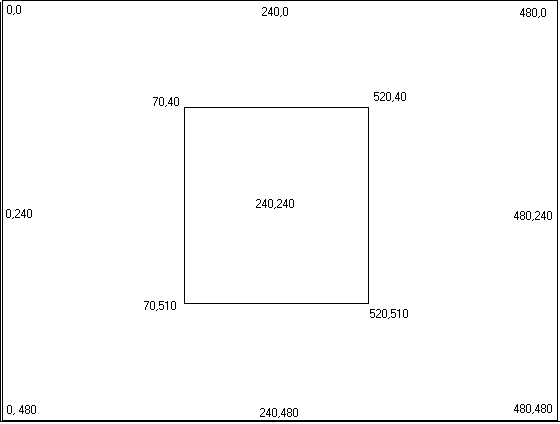Most likely, the pixel coordinates you will be converting from will start at 0,0 in the top left corner, and go to pictureSize, pictureSize in the bottom right. For this illustration we will use (0 to 480 by 0 to 480). The four variables maxX, maxY, minX, minY are parameters to the Mandelbrot generator and are based on the coordinate system (-1.4 to 1.4 by -1.4i to 1.4i). If one were to map pixels on an X axis with matching argand coordinates on a Y axis, a line like the following would be produced: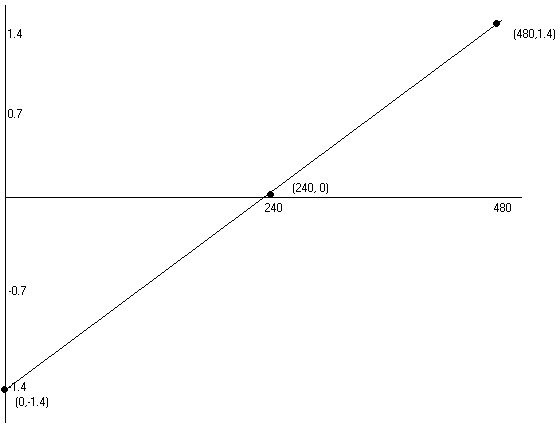Using the equation for a line, (y=mx + b), we have all the ingredients we need. Here are our variables so far:

• y -- the "output". If we can get this number, we can plug it into maxX and run it through the generator.
• m -- the slope of the line. We can find this by (rise/run). From the diagram we see that the slope is (1.4 - -1.4)/(480 - 0). To state this in more general terms, because after zooming we will no longer be using -1.4 to 1.4, and 480 is not the constant pic size, we can change the slope to m = (maxTempX - minTempX)/(pictureSize). maxTempX and minTempY are the OLD values for the max and min of the argand plane. After the user has zoomed in once, the left hand side will no longer be -1.4, so each time he/she zooms, the zoom coordinates must be stored.
• b -- the y intercept. On the diagram, this is -1.4. In more general terms, b = minTempX.
• x --whichever pixel is being converted, which in the maxX case, x = snapShotRect.right. The right most point on the snapshot rect in reference to the pixel plane.

Summarizing, we have:
m = (maxTempX - minTempX) / (m_pictureSize)
b = minTempX
x =snapShotRect.right

Putting this all together into y = mx + b, we get

Y      =                                          M                               *           X               +              B
m_maxX = (( ((maxTempX - minTempX) / (m_pictureSize)) * snapShotRect.right ) + minTempX );

And wala! we can now convert from pixel (X : snapShotRect.right) to argand (Y : m_maxX). Following the same rules, we can now figure out maxY, minX, and minY as well.

m_minX = (( ((maxTempX - minTempX) / (pictureSize)) * snapShotRect.left ) + minTempX );
m_maxY = (( ((maxTempY - minTempY) / (pictureSize)) * snapShotRect.bottom ) + minTempY );
m_minY = (( ((maxTempY - minTempY) / (pictureSize)) * snapShotRect.top ) + minTempY );